Exercises

# Number Sense 3

Understanding how our ten number system works enables students to see interesting and useful patterns and relationships in these math activities. These patterns allow students to develop more efficient mental math strategies when multiplying by multiples of 10 or 100. Students will also review rounding and apply this skill to larger numbers - up to 100,000’s place.
This lesson includes 5 printable learning activities.

## Find the Factors of Prime and Composite Numbers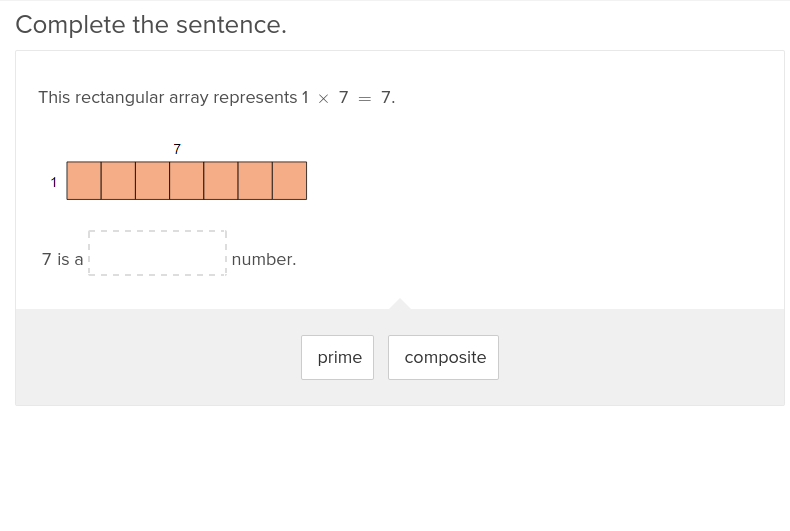Have your fourth graders work through multiplication problems by learning about factors and why they are important for problem solving.

## Place Value and the Thousands Place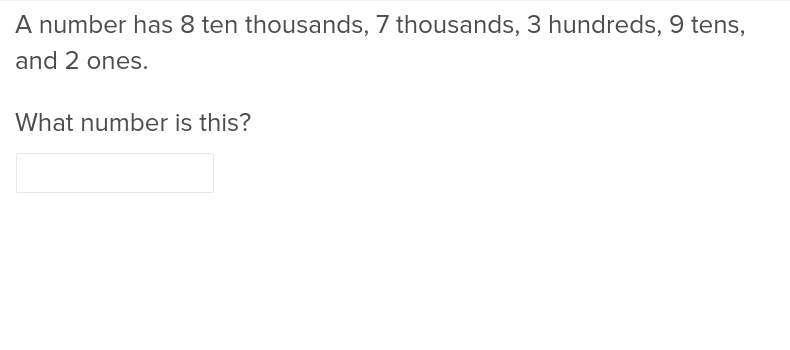Before students can soar in math, they’ll first need to learn how to find the thousands place in this exercise.

## Floyd Danger: Comparing Multi-Digit Numbers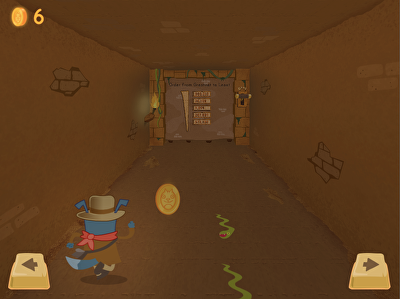Floyd Danger needs to get to the Book of Largest Numbers! In this interactive game, kids navigate through a long and mysterious tunnel and complete math challenges to retrieve his treasure. Along the way, Floyd Danger needs to avoid obstacles and collect tokens. Kids will practice ordering and comparing multi-digit numbers as they go on this adventure.

## Comparing Multi-Digit Numbers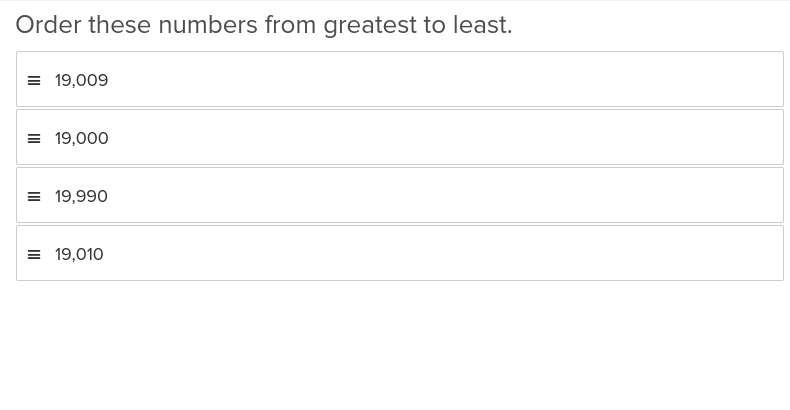This elucidating Education.com exercise will show students working with multiplication, division, addition, or subtraction how to compare multi digit numbers.

## Place Value and Multiplicative Comparisons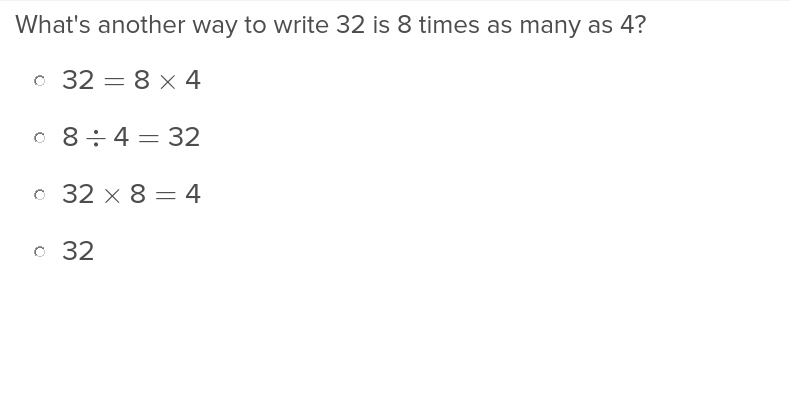Translate word problems into their numeric equivalent with this exercise that teaches both place value and multiplicative comparisons.

## Multiply by Multiples of Ten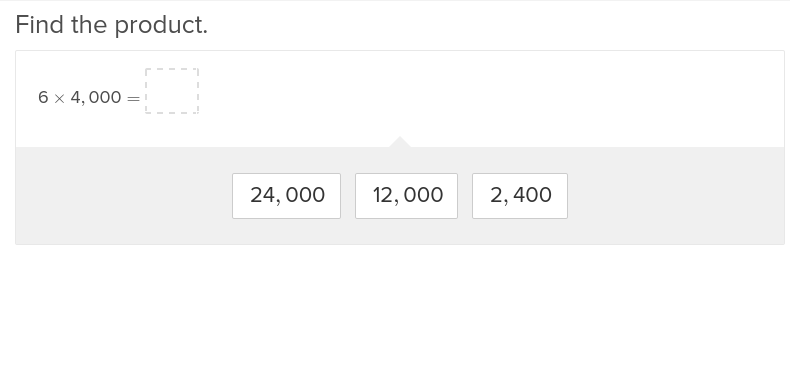Teach fourth graders tricks and tips to multiplying by multiples of ten with these exercises that allow students to work independently.

## Place Value Up to Hundred Thousands Place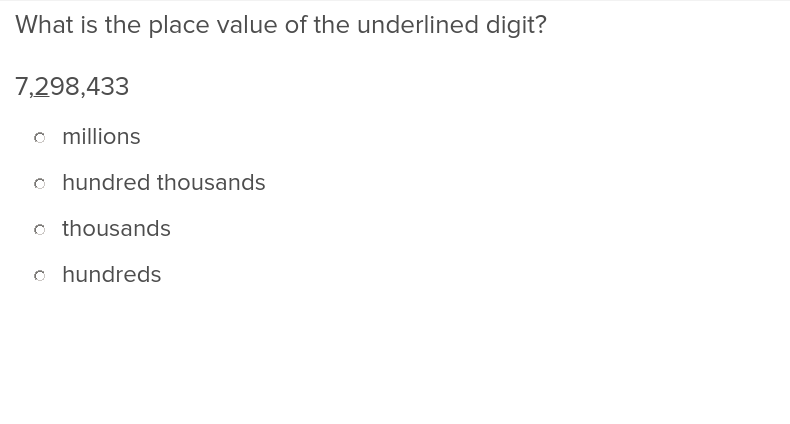Students working with larger numbers will appreciate this exercise that helps them keep numbers straight all the way up to the hundred thousands place.

## Place Value Up to Ten Thousands Place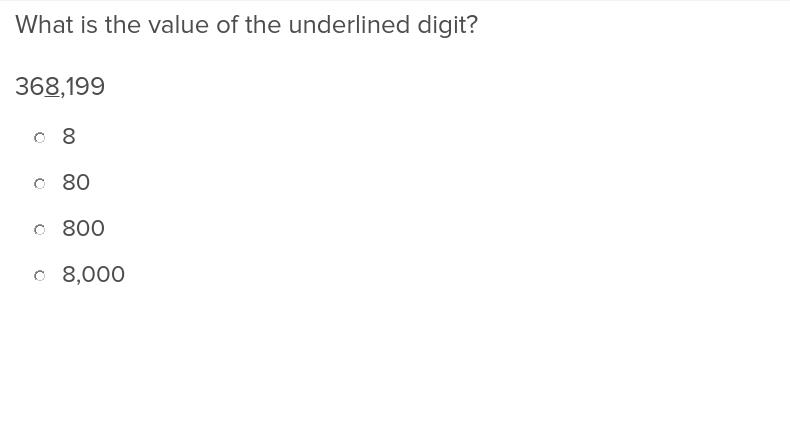Build students’ confidence in solving math problems with large numbers using this exercise on place values up to the ten thousands place.

## Multiplying by 100s: Moving Truck Multiplication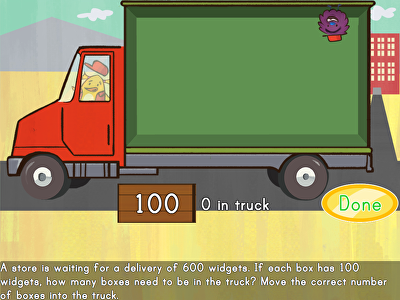Cargo is coming in! Help Birdee make sure that these orders are correct by loading widgets in this transportation math game. Kids find missing factors in multiplication problems by moving the correct number of manipulatives—in groups of 100—on to each truck. Perfect for mastering three-digit multiplication, kids learn how to use patterns when multiplying different numbers by 100.

## Place Value and Numbers in Expanded Form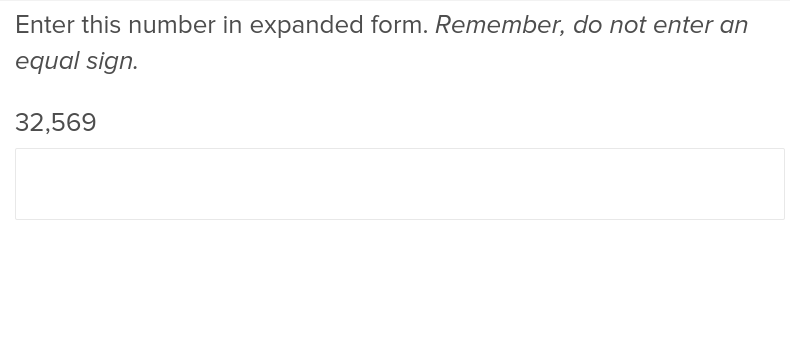This exercise demonstrates how addition in larger numbers is simpler when numbers are dealt with in their expanded form.

## Rounding Multi-Digit Numbers to the Nearest 1,000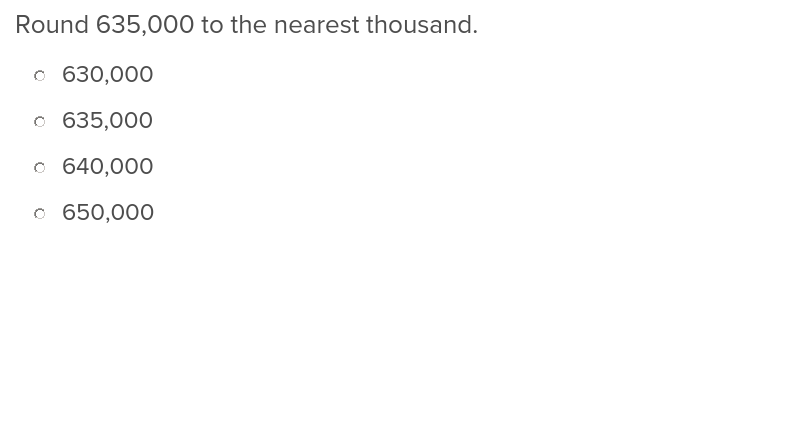Help students simplify math problems with the ability to round multi digit numbers to the nearest 1,000.

## Rounding Multi-Digit Numbers to the Nearest 10000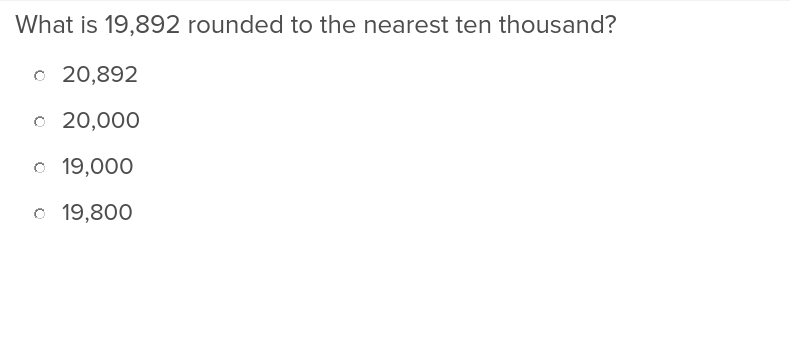Students will find math so much easier after they learn they can round multi digit numbers to the nearest 10,000.

## Rounding Multi-Digit Numbers to the Nearest 100,000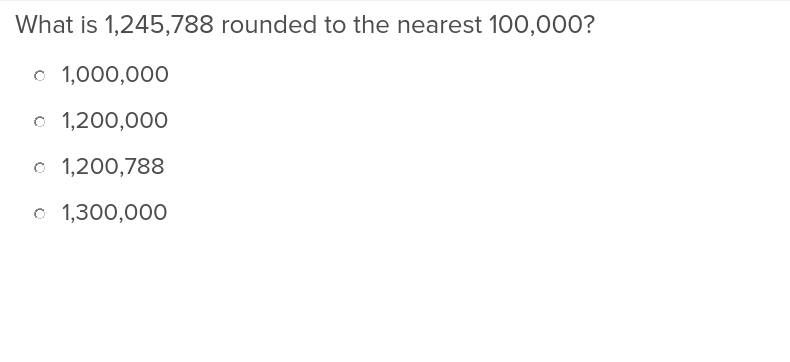With this exercise, students will be able to simplify large numbers by rounding to the nearest 100,000.

## Comparing Decimal Numbers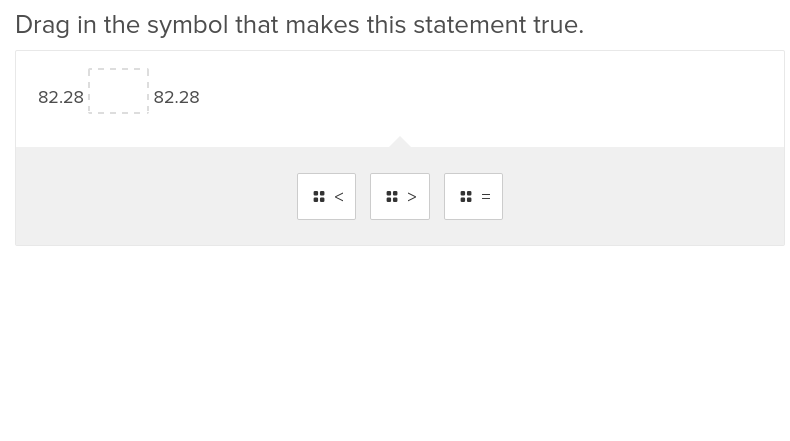Students will gain a solid foundation in comparing decimal numbers by working through this exercise.

## Ordering Decimal Numbers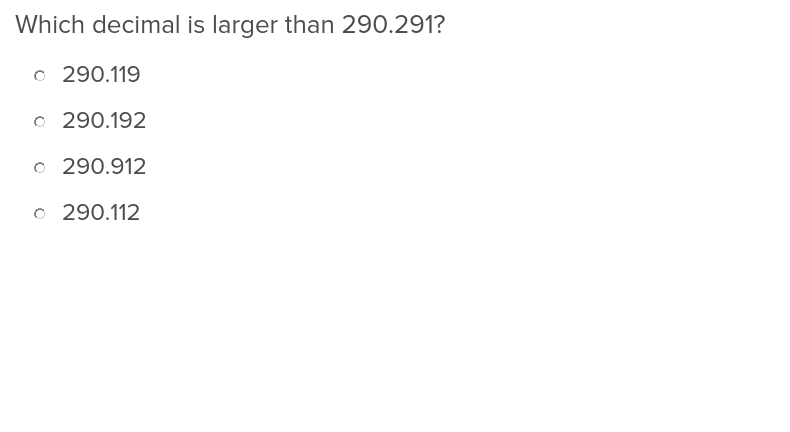This easy and educational exercise from Education.com will teach students how to sort and order decimals.